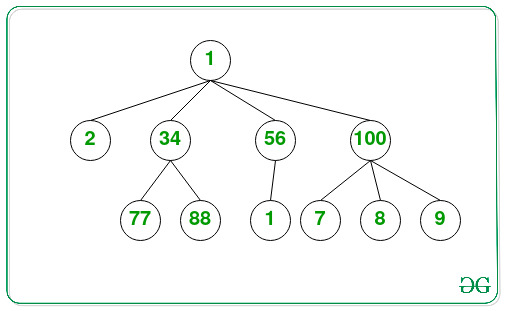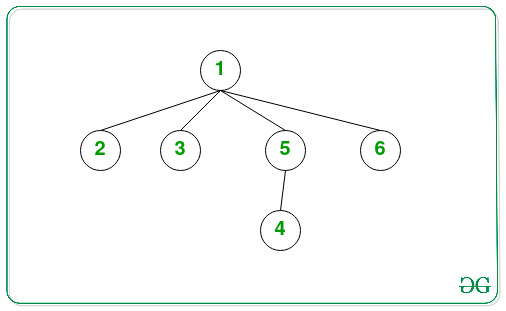Related Articles

# Kth largest element in an N-array Tree

• Difficulty Level : Basic
• Last Updated : 01 Jul, 2021

Given an N-array Tree consisting of N nodes and an integer K, the task is to find the Kth largest element in the given N-ary Tree.

Examples:

Input: K = 3Output: 77
Explanation:
The 3rd largest element in the given N-array tree is 77.

Input: K = 4Output: 3

Approach: The given problem can be solved by finding the largest element in the given range for K number of times and keep updating the end of the range to the largest element found so far. Follow the steps below to solve the problem:

• Initialize a variable say, largestELE as INT_MIN.
• Define a function, say largestEleUnderRange(root, data), and perform the following steps:
• If the value of the current root is less than the data, then update the value of largestELe as the maximum of largestELe and the current root’s value.
• Iterate over all children of the current root and recursively call for the function largestEleUnderRange(child, data).
• Initialize a variable say, ans as INT_MAX to store the Kth largest element.
• Iterate over the range [0, K – 1] recursively call for the function largestEleUnderRange(root, ans) and update the value of ans as largestELe and largestELe as INT_MIN.
• After completing the above steps, print the value of ans as the resultant Kth maximum value.

Below is the implementation of the above approach.

## C++

 `// C++ program for the above approach` `#include ``using` `namespace` `std;` `// Structure of N-array Tree``class` `Node {``public``:``    ``int` `data;``    ``vector childs;``};` `// Stores the minimum element``// in the recursive call``int` `largestELe = INT_MIN;` `// Function to find the largest``// element under the range of key``void` `largestEleUnderRange(``    ``Node* root, ``int` `data)``{``    ``// If the current root's value``    ``// is less than data``    ``if` `(root->data < data) {``        ``largestELe = max(root->data,``                         ``largestELe);``    ``}` `    ``// Iterate over all the childrens``    ``for` `(Node* child : root->childs) {` `        ``// Update under current range``        ``largestEleUnderRange(child, data);``    ``}``}` `// Function to find the Kth Largest``// element in the given N-ary Tree``void` `KthLargestElement(Node* root,``                       ``int` `K)``{``    ``// Stores the resultant``    ``// Kth maximum element``    ``int` `ans = INT_MAX;` `    ``// Iterate over the range [0, K]``    ``for` `(``int` `i = 0; i < K; i++) {` `        ``// Recursively call for``        ``// finding the maximum element``        ``// from the given range``        ``largestEleUnderRange(root, ans);` `        ``// Update the value of``        ``// ans and largestEle``        ``ans = largestELe;``        ``largestELe = INT_MIN;``    ``}` `    ``// Print the result``    ``cout << ans;``}` `// Function to create a new node``Node* newNode(``int` `data)``{``    ``Node* temp = ``new` `Node();``    ``temp->data = data;` `    ``// Return the created node``    ``return` `temp;``}` `// Driver Code``int` `main()``{``    ``/*   Create below the tree``     ``*              10``     ``*        /   /    \   \``     ``*        2  34    56   100``     ``*       / \         |   /  | \``     ``*      77  88       1   7  8  9``     ``*/` `    ``Node* root = newNode(10);``    ``(root->childs).push_back(newNode(2));``    ``(root->childs).push_back(newNode(34));``    ``(root->childs).push_back(newNode(56));``    ``(root->childs).push_back(newNode(100));``    ``(root->childs->childs).push_back(newNode(77));``    ``(root->childs->childs).push_back(newNode(88));``    ``(root->childs->childs).push_back(newNode(1));``    ``(root->childs->childs).push_back(newNode(7));``    ``(root->childs->childs).push_back(newNode(8));``    ``(root->childs->childs).push_back(newNode(9));` `    ``int` `K = 3;``    ``KthLargestElement(root, K);` `    ``return` `0;``}`

## Javascript

 ``
Output:
`77`

Time Complexity: O(N*K)
Auxiliary Space: O(1)

Attention reader! Don’t stop learning now. Get hold of all the important DSA concepts with the DSA Self Paced Course at a student-friendly price and become industry ready.  To complete your preparation from learning a language to DS Algo and many more,  please refer Complete Interview Preparation Course.

In case you wish to attend live classes with experts, please refer DSA Live Classes for Working Professionals and Competitive Programming Live for Students.

My Personal Notes arrow_drop_up